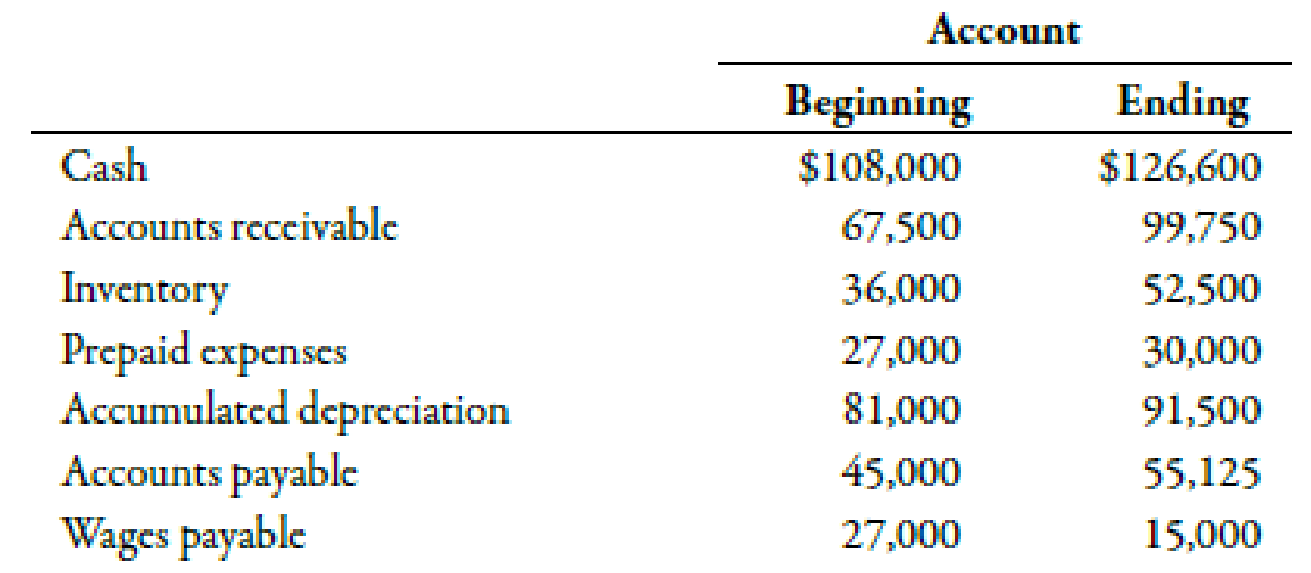Chapter 14, Problem 36E### Managerial Accounting: The Corners...

7th Edition
Maryanne M. Mowen + 2 others
ISBN: 9781337115773

#### Solutions

Chapter
Section### Managerial Accounting: The Corners...

7th Edition
Maryanne M. Mowen + 2 others
ISBN: 9781337115773
Textbook Problem
81 views

# During the year, Hepworth Company earned a net income of $61,725. Beginning and ending balances for the year for selected accounts are as follows:There were no financing or investing activities for the year. The above balances reflect all of the adjustments needed to adjust net income to operating cash flows.Required: 1. Prepare a schedule of operating cash flows using the indirect method. 2. Suppose that all the data are used in Requirement 1 except that the ending accounts payable and cash balances are not known. Assume also that you know that the operating cash flow for the year was$20,475. What is the ending balance of accounts payable? 3. CONCEPTUAL CONNECTION Hepworth has an opportunity to buy some equipment that will significantly increase productivity. The equipment costs $25,000. Assuming exactly the same data used for Requirement 1, can Hepworth buy the equipment using this year’s operating cash flows? If not, what would you suggest be done? 1. To determine Construct a schedule showing the operating cash flows with the use of indirect method. Explanation Cash Flows from Operating Activities: This category of a cash flow statement shows the operational and profit generating activities in a firm. The operating cash flows increase or decrease the current assets and current liabilities of a firm. The schedule showing operating cash flows using indirect method is in the table below:  H Company Schedule for Cash Flow from Operating Activities For the year ended December 31, 20X1 Particulars Amount ($) Cash flows from operating activities: Net income 61,725 Add/ Less: Increase in accounts receivable1 (32,250) Increase in inventory2 (16,500) Increase in prepaid expenses3 (3,000) Increase in accounts payable4 10,125 Decrease in wages payable5 (12,000) Depreciation expense6 10,500 Net cash from operating activities 18,600

Table (1)

Therefore, net cash flow from operating activities is $18,600. Working Note: 1. Calculation of difference in accounts receivable: (DifferenceinAccountsReceivable)=(EndingAccountsReceivableBeginningAccountsReceivable)=($99,750$67,500)=$32,250

2.

Calculation of difference in amount of inventories:

(DifferenceinInventories)=(EndingInventoriesBeginningInventories)=($52,500$36,000)=$16,500 3. Calculation of difference in prepaid expenses: (DifferenceinPrepaidExpenses)=(EndingPrepaidExpensesBeginningPrepaidExpenses)=($30,000$27,000)=$3,000

4

2.

To determine

Compute the ending balance of accounts payable.

3.

To determine

Explain whether Company H can buy the equipment using the current year operating cash flows. Also, give suggestions if the company is not able to buy the equipment.

### Still sussing out bartleby?

Check out a sample textbook solution.

See a sample solution

#### The Solution to Your Study Problems

Bartleby provides explanations to thousands of textbook problems written by our experts, many with advanced degrees!

Get Started